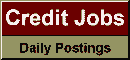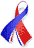DefaultRisk.com the web's biggest credit risk modeling resource.Export citation to:- HTML- Text (plain)- BibTeX- RIS- ReDIF

Neftci, Salih N., "Value at Risk Calculations, Extreme Events, and Tail Estimation", Journal of Derivatives, Vol. 7, (Spring 2000), pp. 23-38.

Abstract: The notion of extreme movements in asset prices is implicit in current risk management practices. Capital adequacy assumes a threshold that classifies observed changes in market risk factors either as extreme or ordinary. A probability is first chosen to measure the "extremeness" of events that may affect a particular portfolio. This probability then determines the proper threshold.

Underlying this approach is the central limit theorem, which yields an asymptotic normal distribution for the risk factors under consideration. If it is possible to obtain an asymptotic distribution for all possible values of risk factors, however, why not do the same for extreme observations as well? Extremal theory does exactly this, and provides a methodology that can be used to make statistical inferences on extremes only, using a new set of tools.

The value at risk calculations provided here use extreme distribution theory and yield some remarkable results. Both the in-sample and out-of-sample data show that extreme distribution theory performs surprisingly well in capturing both the rate of occurrence and the extent of extreme events in financial markets. In fact, statistical theory of extremes appears to be a more natural and robust approach to risk management calculations.

Books Referenced in this paper:  (what is this?)